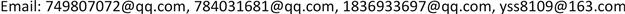1. 引言

2. 现有研究成果对比分析

3. 人脸检测

3.1. Haar分类器算法

Opencv自带了haar分类器，Haar分类器算法的要点如下：

· 使用Haar-like特征做检测。

· 使用积分图(Integral Image)对Haar-like特征求值进行加速。

· 使用筛选式级联把强分类器级联到一起，提高准确率。

1) 给定训练样本集S，共N个样本，其中X和Y分别对应于正样本和负样本；T为训练的最大循环次数；

2) 初始化样本权重为1/N，即为训练样本的初始概率分布；

3) 第一次迭代训练N个样本，得到第一个最优弱分类器;

4) 提高上一次迭代中被误判的样本的权重；

5) 将新的样本和上次分错的样本放在一起进行新一轮的训练;

6) 循环执行4~5步骤，T轮后得到T个最优弱分类器。

7) 将T个最优弱分类器组合而得强分类器，组合方式(1)所示：

C ( x ) = { 1 ∑ t = 1 T α t h t ( x ) ≥ 1 2 ∑ t T α t 0 其 他 (1)

C FINAL ( x ) = sin ( ∑ i = 1 M a i C i ( x ) ) (2)

4. 人脸图像预处理

4.1. 图像去噪

SNN均值滤波算法

4.2. 图像增强

1) 求出原图 [ f ( x , y ) ] M × N 的灰度直方图，用256维的向量h表示。则

h ( x ) = p ( x ) = n k N     ( k = 0 , 1 , ⋯ , L − 1 )

2) 由直方图均衡化映射函数进行直方图的均衡化。则映射函数为：

g ( x , y ) = { ( L − 1 ) ,       ∑ k = 0 f ( x , y ) p ( x ) f ( x , y ) ≠ 0 0 ,                         f ( x , y ) = 0

4.3. 图像分割

1) 对待分割的图像 [ f ( x , y ) ] m × n 按照某锐化算子(如sobel算子)求梯度图 ∇ [ f ( x , y ) ] m × n 。

2) 按照如下公式求梯度图 ∇ [ f ( x , y ) ] M × N 的加权灰度直方图 h ( k ) ( k = 0 , 1 , 2 , ⋯ , 255 ) ，即

h ( k ) = w ( k ) ⋅ N ( k ) ,     k = 0 , 1 , 2 , ⋯ , 255

3) 将加权梯度直方图，带入最大熵阈值方法，求出 ∇ [ f ( x , y ) ] m × n 的分割阈值。

5. 人脸识别

1) 将X的每一行(代表一个属性字段)进行零均值化，即减去这一行的均值，使每一维的均值为0。即

X [ n , m ] = X [ n , m ] − 1 m ∑ 1 m X [ n , m ] (1)

2) 求出协方差：数学上可以用两个字段的协方差表示其相关性，由于已经让每个字段均值为0，则：

C o v ( a , b ) = 1 m ∑ i = 1 m a i b i (2)

3) 协方差矩阵：

C = 1 m ∑ i = 1 m ( X i − X ¯ ) ( X i − X ¯ ) T = 1 m X ⋅ X T (3)

4) 求出协方差矩阵的特征值及对应的特征向量。

5) 将特征向量按对应特征值大小从上到下按行排列成矩阵，取前k行组成矩阵P。

6) Y = PXY = PX即为降维到k维后的数据。

6. 移动端设计与实现6.1. 系统设计 [<xref ref-type="bibr" rid="hanspub.24235-ref8">8</xref>]

6.2. 系统主要功能

6.3. 技术选型6.3.1. 服务端

6.3.2. 数据库

6.4. 效果展示

7. 结语